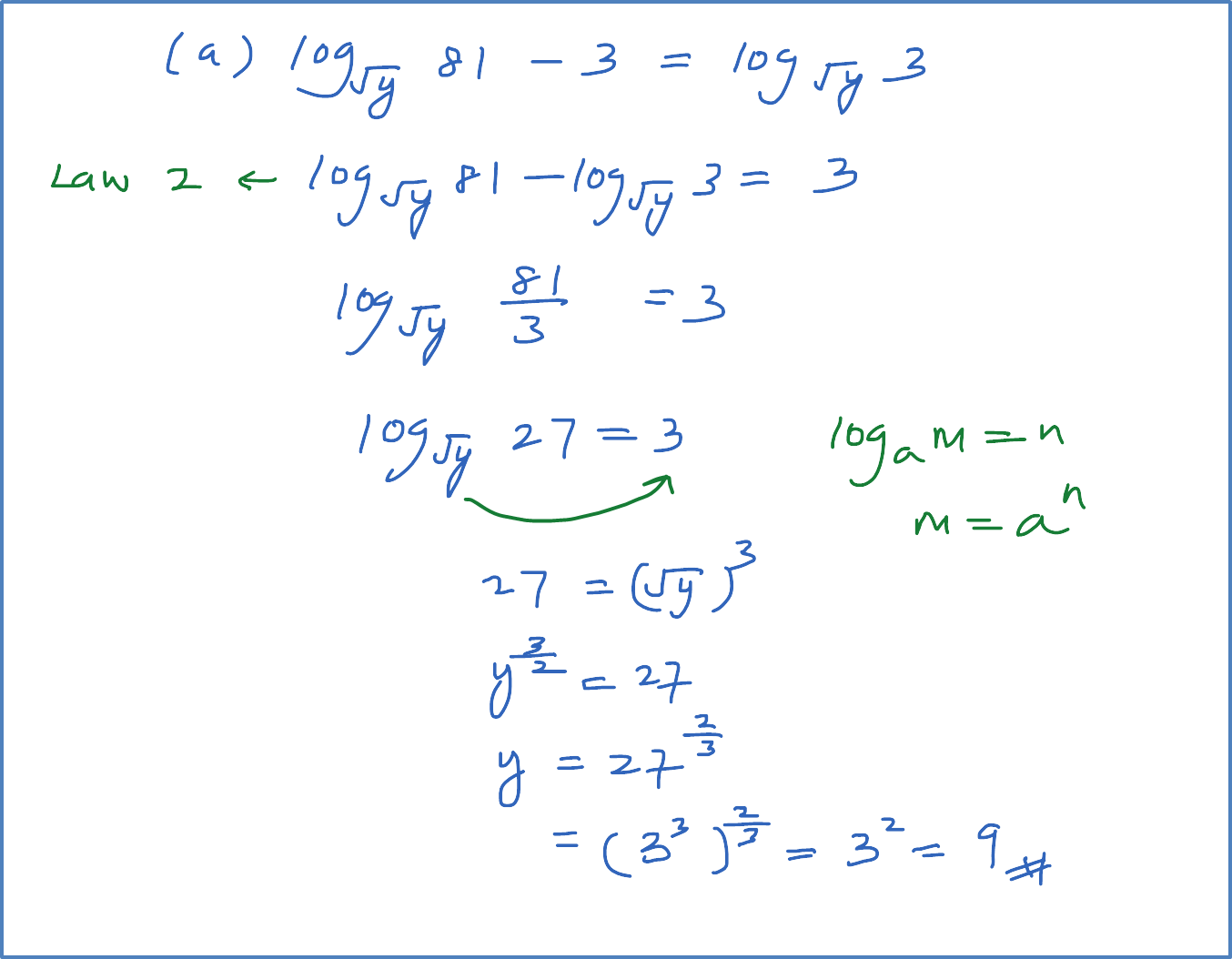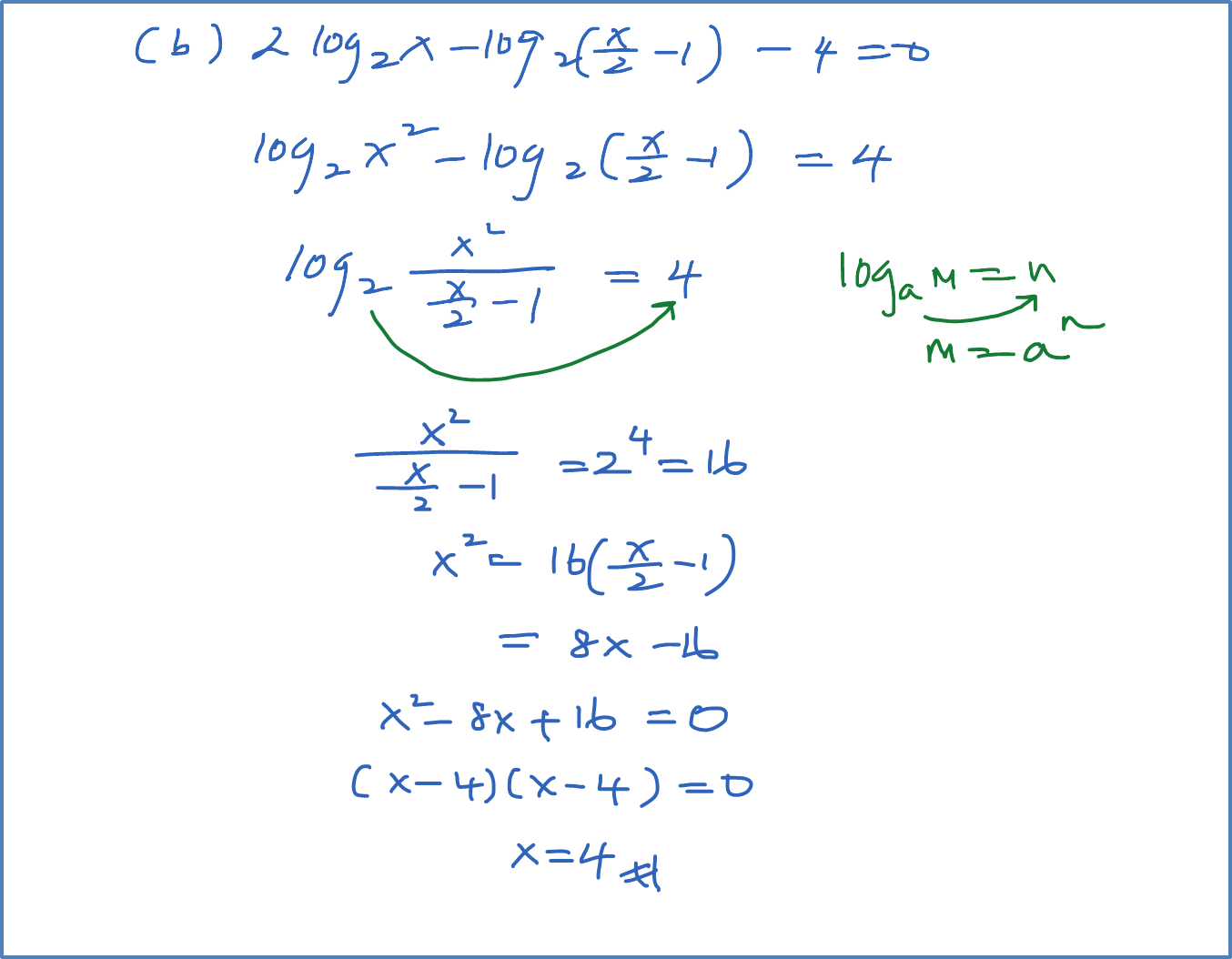# 5.4.2 Logarithms Equation – Example 2

Example 2
Solve the following equation:
(a) ${\mathrm{log}}_{\sqrt{y}}81-3={\mathrm{log}}_{\sqrt{y}}3$
(b) $2{\mathrm{log}}_{2}x-{\mathrm{log}}_{2}\left(\frac{x}{2}-1\right)-4=0$